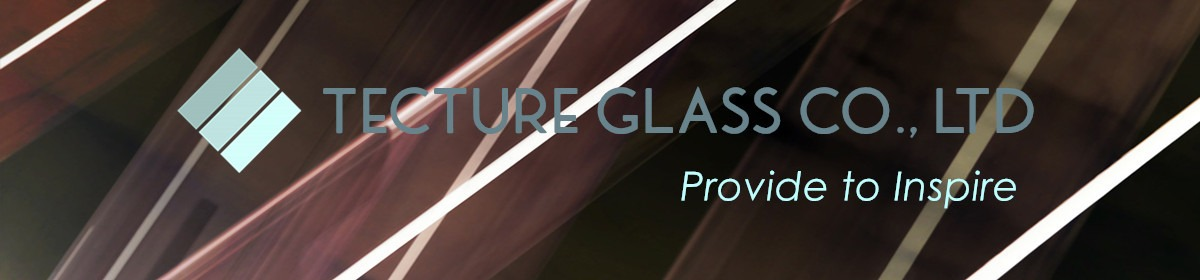• Sourcing solutions
• Services & Membership
• Help
Orders 0
Favorites 0
Guangzhou Tecture Glass Co., Ltd.### Featured Products

Anti slip glass
Digital printed glass
Specialty laminated glass
SGP Laminated glass
\$100.00 - \$185.00/square meter
1 square meter(Min. Order)
\$100.00 - \$185.00/square meter
1 square meter(Min. Order)
\$100.00 - \$185.00/square meter
1.0 square meter(Min. Order)
\$100.00 - \$185.00/piece
1 piece(Min. Order)
\$100.00 - \$185.00/piece
1 piece(Min. Order)
\$100.00 - \$185.00/piece
1 piece(Min. Order)
\$90.00 - \$120.00/square meter
1 square meter(Min. Order)
\$90.00 - \$120.00/square meter
1 square meter(Min. Order)
\$90.00 - \$120.00/square meter
1 square meter(Min. Order)
\$90.00 - \$120.00/square meter
1.0 square meter(Min. Order)
\$90.00 - \$120.00/square meter
1.0 square meter(Min. Order)
\$85.00 - \$180.00/square meter
1.0 square meter(Min. Order)
\$180.00 - \$250.00/square meter
1 square meter(Min. Order)
\$198.00 - \$304.00/square meter
1.0 square meter(Min. Order)
\$112.00 - \$200.00/square meter
1.0 square meter(Min. Order)
\$135.00 - \$205.00/square meter
1.0 square meter(Min. Order)
\$135.00 - \$205.00/square meter
1.0 square meter(Min. Order)
\$135.00 - \$205.00/square meter
1.0 square meter(Min. Order)

### Products Category

\$45.00 - \$55.00/square meter
1.0 square meter(Min. Order)
\$68.00 - \$150.00/square meter
1 square meter(Min. Order)
\$68.00 - \$150.00/square meter
1.0 square meter(Min. Order)
\$53.00 - \$70.00/piece
1 piece(Min. Order)
\$198.00 - \$304.00/square meter
1.0 square meter(Min. Order)
\$198.00 - \$304.00/square meter
1.0 square meter(Min. Order)
\$198.00 - \$304.00/square meter
1.0 square meter(Min. Order)

### SPECIALTY LAMINATED GLASS

\$85.00 - \$180.00/square meter
1.0 square meter(Min. Order)
\$85.00 - \$180.00/square meter
1.0 square meter(Min. Order)
\$180.00 - \$250.00/square meter
1.0 square meter(Min. Order)
\$198.00 - \$304.00/square meter
1.0 square meter(Min. Order)
\$180.00 - \$250.00/square meter
1.0 square meter(Min. Order)
\$224.00 - \$304.00/square meter
1.0 square meter(Min. Order)
\$224.00 - \$304.00/square meter
1.0 square meter(Min. Order)
\$198.00 - \$304.00/square meter
1.0 square meter(Min. Order)

### PATTERNED GLASS

\$55.00 - \$80.00/square meter
1.0 square meter(Min. Order)
\$45.00 - \$55.00/square meter
1.0 square meter(Min. Order)
\$45.00 - \$55.00/square meter
1 square meter(Min. Order)
\$45.00 - \$55.00/square meter
1.0 square meter(Min. Order)
\$45.00 - \$55.00/square meter
1.0 square meter(Min. Order)

### Products Category

\$95.00 - \$185.00/square meter
1.0 square meter(Min. Order)
\$90.00 - \$120.00/square meter
1.0 square meter(Min. Order)
\$198.00 - \$304.00/square meter
1 square meter(Min. Order)
\$45.00 - \$55.00/square meter
1.0 square meter(Min. Order)
\$45.00 - \$55.00/square meter
1.0 square meter(Min. Order)
\$55.00 - \$80.00/square meter
1.0 square meter(Min. Order)